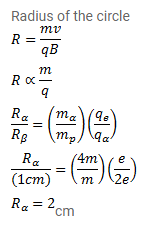# A proton describes a circle of radius 1cm

Question:

A proton describes a circle of radius $1 \mathrm{~cm}$ in a magnetic field of strength $0.10 \mathrm{~T}$. What would be the radius of the circle described by an $\alpha$ - particle moving with the same speed in the same magnetic field?

Solution: